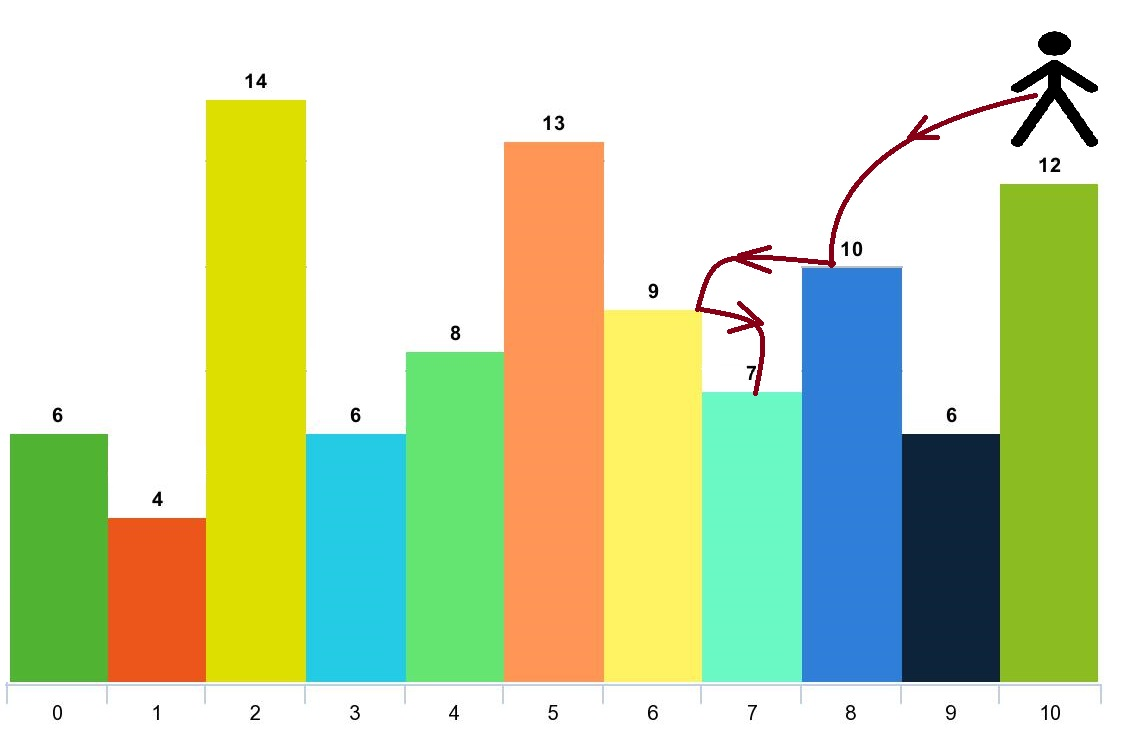# 跳跃游戏 V

## 1340. 跳跃游戏 V (Hard)

• `i + x` ，其中 `i + x < arr.length` 且 `0 < x <= d` 。
• `i - x` ，其中 `i - x >= 0` 且 `0 < x <= d` 。```输入：arr = [6,4,14,6,8,13,9,7,10,6,12], d = 2

```

```输入：arr = [3,3,3,3,3], d = 3

```

```输入：arr = [7,6,5,4,3,2,1], d = 1

```

```输入：arr = [7,1,7,1,7,1], d = 2

```

```输入：arr = , d = 1

```

• `1 <= arr.length <= 1000`
• `1 <= arr[i] <= 10^5`
• `1 <= d <= arr.length`

[动态规划]